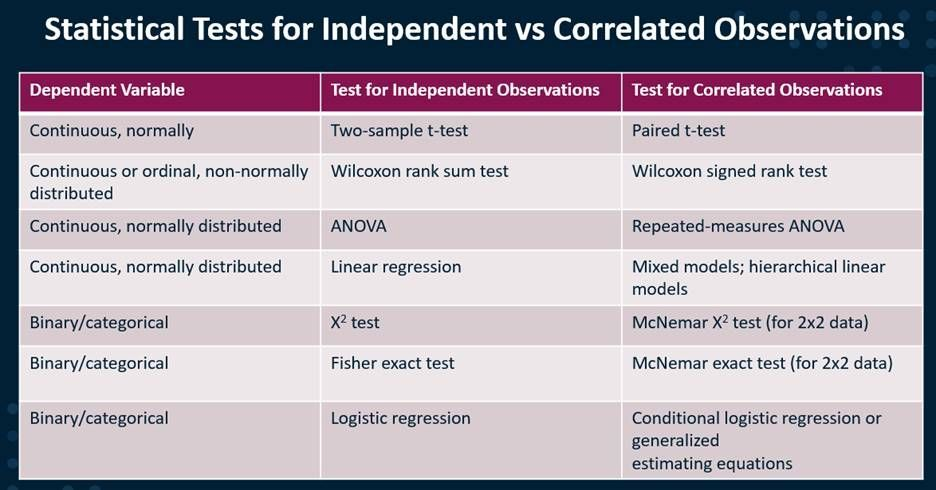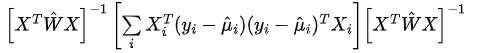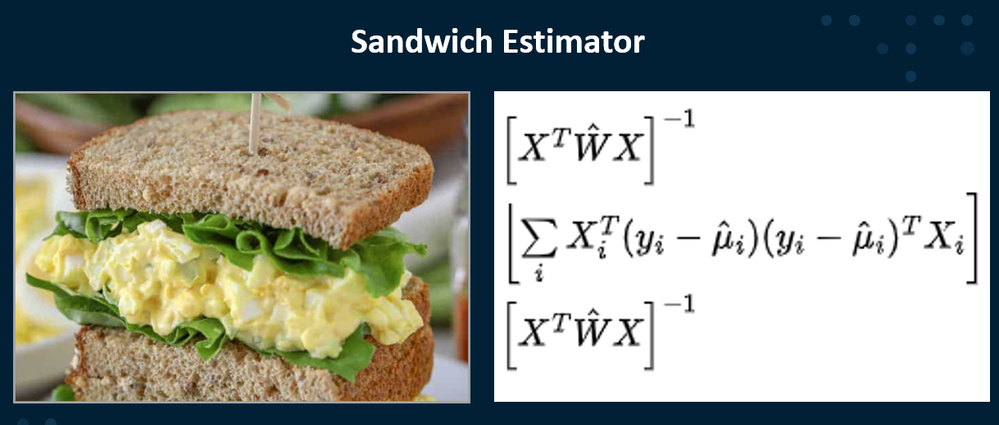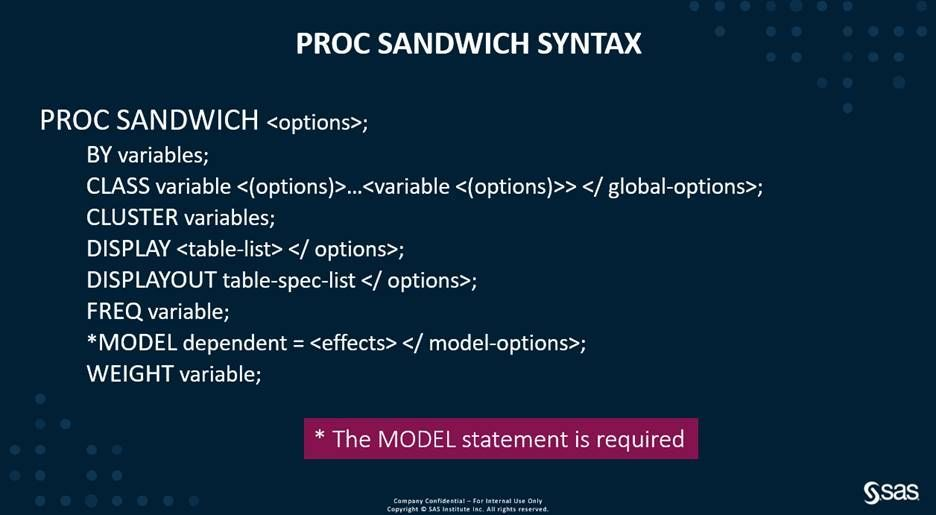## Using the SAS Sandwich Procedure with Large Correlated Datasets

Started ‎12-09-2021 by
Modified ‎12-09-2021 by
Views 3,055

Background

PROC SANDWICH can help you deal with large correlated datasets.  Large datasets with many correlated observations can occur in many domains, such as education research, genetics, health and life sciences, geospatial and others. Correlated data result when pairs or clusters of observations are more similar to each other than to other observations in the data set. One example is measures on the same individual or on the same family. A classic example of a source of correlated observations is repeated measures studies. Another example is cluster randomized trials. Cluster randomized trials assign interventions to groups of subjects (e.g., schools, clinics) rather than to individuals.Correlated observations present difficulties because the covariance matrix for their observations is not diagonal. Note that correlated observations is different from correlated input variables.

Correlated Observations: Correlated observations might be repeated measures of diastolic blood pressure. Mark’s diastolic blood pressure in May is not independent of Mark’s diastolic blood pressure in January.

Correlated Input Variables: Diastolic blood pressure and systolic blood pressure might be two different input variables. Mark’s diastolic blood pressure is likely to be correlated with his systolic blood pressure.

Correlation and covariance matrices can be easily created in SAS Studio:

``````***LOAD data from SASHELP***;
cas mysession sessopts=(caslib=casuser timeout=1800 locale=”en_US”);
libname casuser cas caslib=”casuser”;
proc casutil;
droptable casdata=”casuser.class” quiet;
casout=”CLASS” promote;
run;
quit; *best practice is to close the CAS thread;

***Use PROC CORR to get the covariance matrix and Pearson Correlation Coefficients***;
ods select Cov PearsonCorr;
proc corr data=casuser.class noprob outp=OutCorr /** store results **/
nomiss /** listwise deletion of missing values **/
cov; /** include covariances **/
var Height Weight Age;
run;``````

Correlations are just a standardized form of covariance. To calculate the Pearson's correlation coefficient, you divide the covariance of the two variables by the product of their standard deviations. This effectively removes the units, creating a unitless measure that ranges from -1 and 1.

For the record, both correlated observations and correlated input variables cause issues with many statistical methods. This is because many statistical tests, such as ordinary least squares regression, assume that observations are independent. Applying these tests to correlated observations can lead to overestimating and underestimating p-values.

Here we will focus on correlated observations. One solution for dealing with these correlated data is to change your statistical methods.Select any image to see a larger version.
Mobile users: To view the images, select the "Full" version at the bottom of the page.

Another solution is using a robust variance estimator such as the Sandwich Estimator.

Sandwich Estimator

The ideas behind the Sandwich Estimator were first proposed in 1967 by Peter Huber, a Swiss statistician of the Eidgenössische Technische Hochschule Zürich. Kung-Yee Liang and Scott Zeger of The Johns Hopkins University in Baltimore, Maryland, USA took the Sandwich Estimator and applied it to longitudinal data in 1986.

It is called a Sandwich Estimator because it is calculated as the product of three matrices:The first layer is the model-based variance (the bread). It is multiplied by the middle matrix. The meat (or peanut butter for my vegan friends) is the middle matrix. This middle matrix is multiplied by the last layer, also a model-based variance matrix.It turns out that the Sandwich Estimator provides a good estimate of Cov(β-hat) in large samples regardless of the true form of Cov(yi). Be careful with smaller samples, though; one issue might be that your classic 95% intervals obtained by plus/minus 2 standard errors aren’t correct.

Scott Zeger (1988) revealed that the use of independence estimating equations with the Sandwich Estimator can be highly efficient if within-entity correlations are not strong.

PROC SANDWICH

PROC SANDWICH lets you analyze large data with many correlated observations by using a robust variance estimator to adjust for correlation after the model is estimated. Optimization algorithms typically require the solution of many systems of linear equations. With big data, these computations can suck up computer processing time. Sparse matrix techniques such as PROC SANDWICH work to address this issue.See the SAS Viya documentation for detailed code examples.

Find more articles from SAS Global Enablement and Learning here.

Version history
Last update:
‎12-09-2021 11:04 AM
Updated by:Contributors
Article Labels
Article Tags